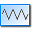Resistor Calculator 1.0.8

Determine the color code of a resistor and calculate resistors for LED circuits.

By using this resistor calculator it is very simple to determine the color code of a resistor based on the resistor value. Of course, you can also provide a color code and the program will show you the resulting resistor value.

On the basis of the entered or calculated resistor value additionally the closest resistor within the E series as well as within the Renard numbers is displayed automatically.

As another feature the program can calculate the required resistor value for LED circuits (single LED, series connection and parallel connection) considering the supply voltage, the voltage drop across the LED(s) as well as the current of the LED(s).

Features of Resistor Calculator at a glance:

• Calculating the color code of a resistor value.
• Calculating the resistor value of a color code.
• Displaying the closest resistor of the E series and Renard numbers.
• Resistor calculation for LED circuits (single, in series, parallel).
• Integrated help system.
• Multilingual (at the moment English and German).
• Intuitive program interface.
• Free e-mail support in case of problems or questions.

This product has been tested on Windows XP, Vista, 7, 8.

If you want you can uninstall Resistor Calculator again at any time using the control panel "Add or Remove Programs" feature.

If you have questions or problems with this software, please simply visit the Forum or just contact us.

Step 1

Click on "Save" to save the application.

Step 2

Click on "Run" to start the application.

Step 3

Now follow easy steps of the setup assistant.

Step 4

Now follow easy steps of the setup assistant.

1. Step 1

Click on the name of the program